CBSE Class 12 Sample Paper for 2022 Boards (For Term 2)

Class 12
Solutions of Sample Papers and Past Year Papers - for Class 12 Boards

## Find ∫1▒〖(x + 1)/((x^2  + 1)  x) dx〗

This question is similar to Ex 13.2, 2 - Chapter 13 Class 12 - Probability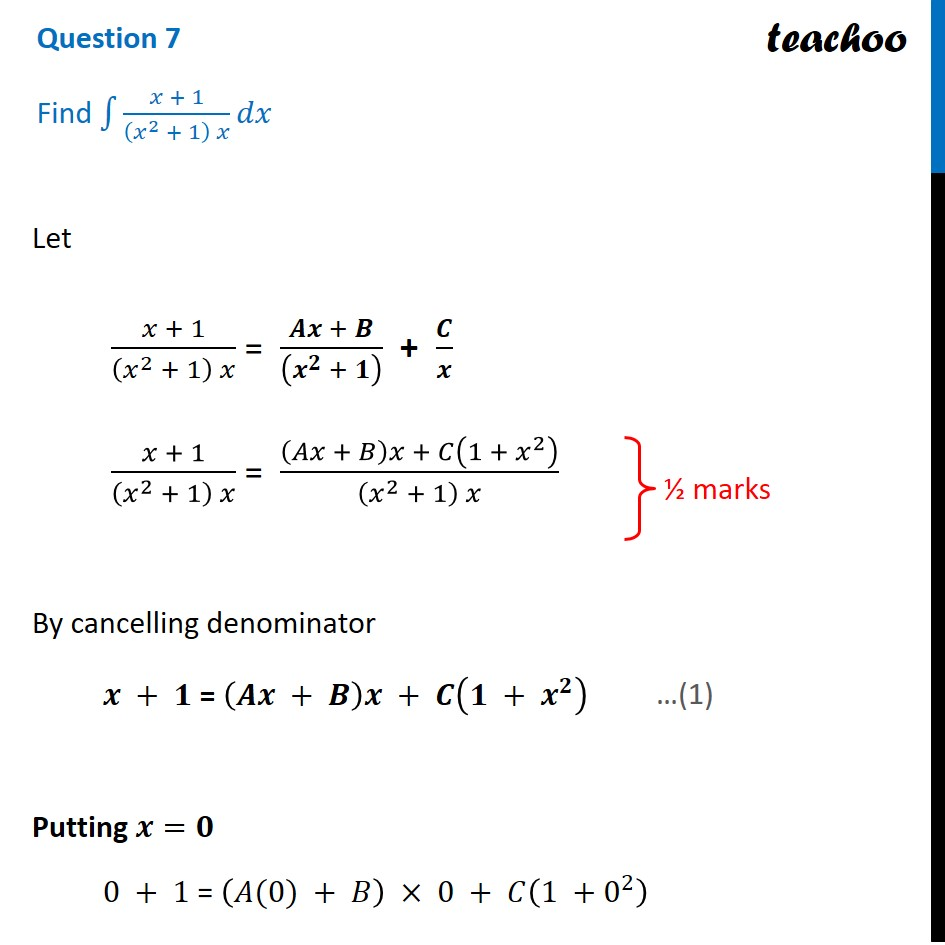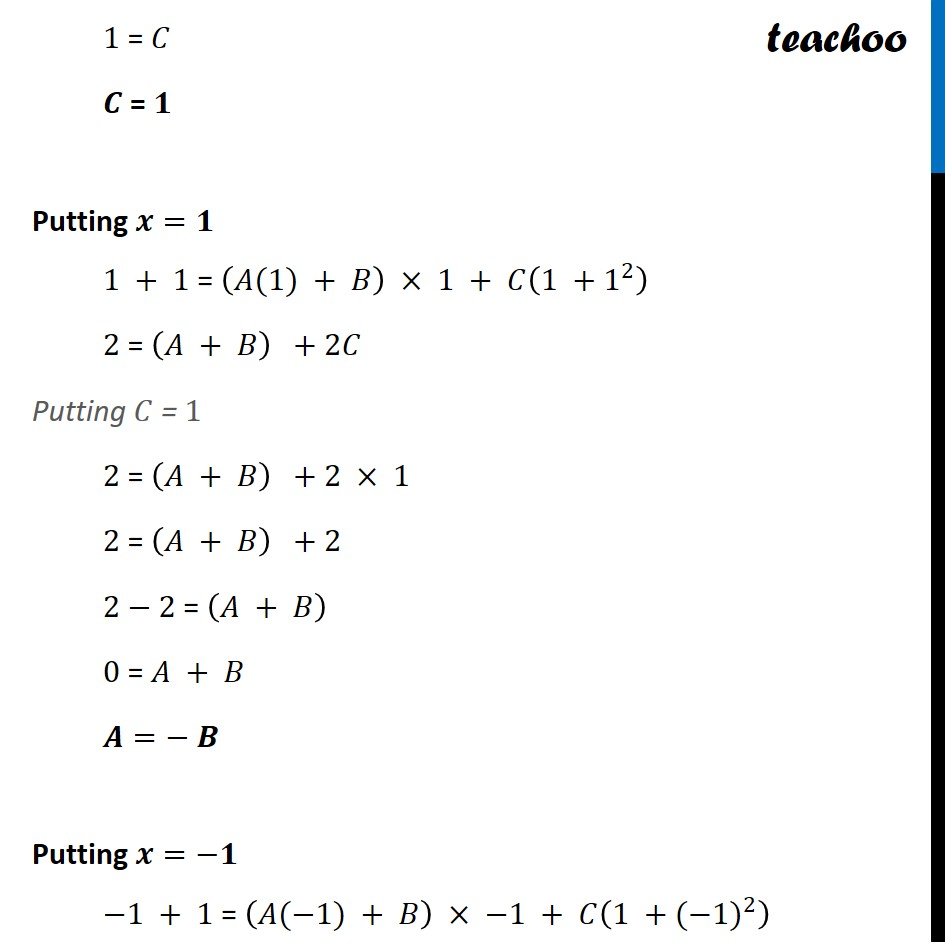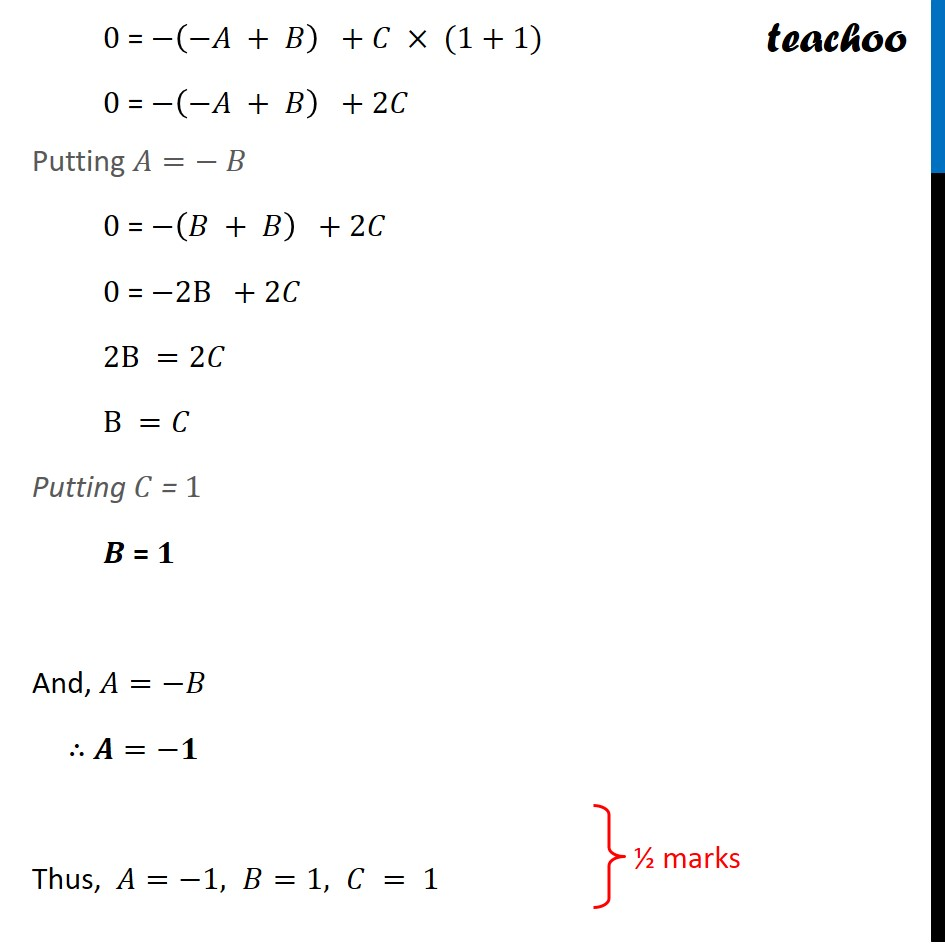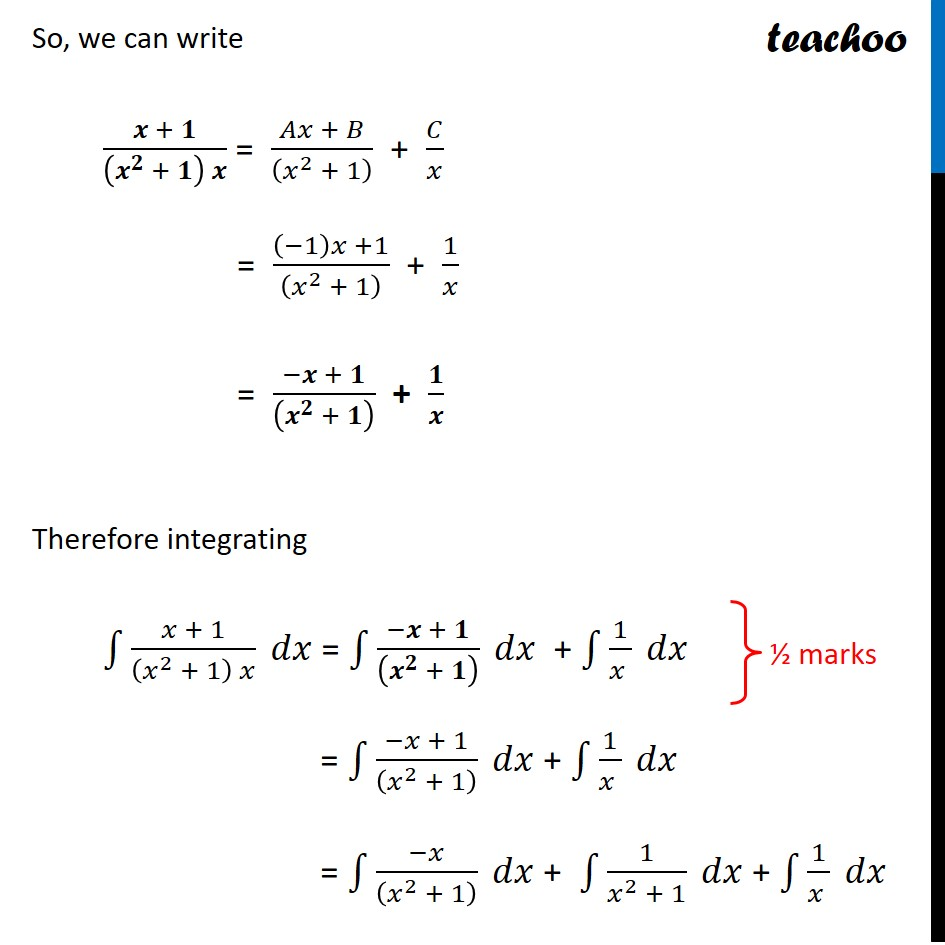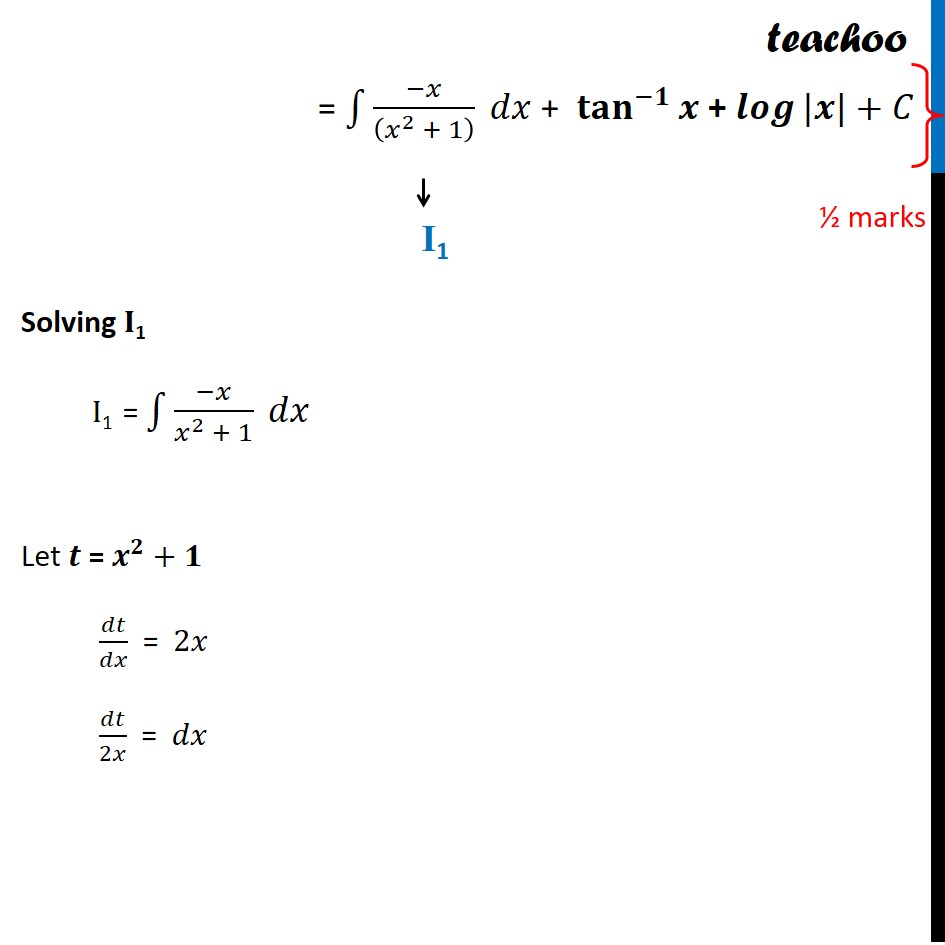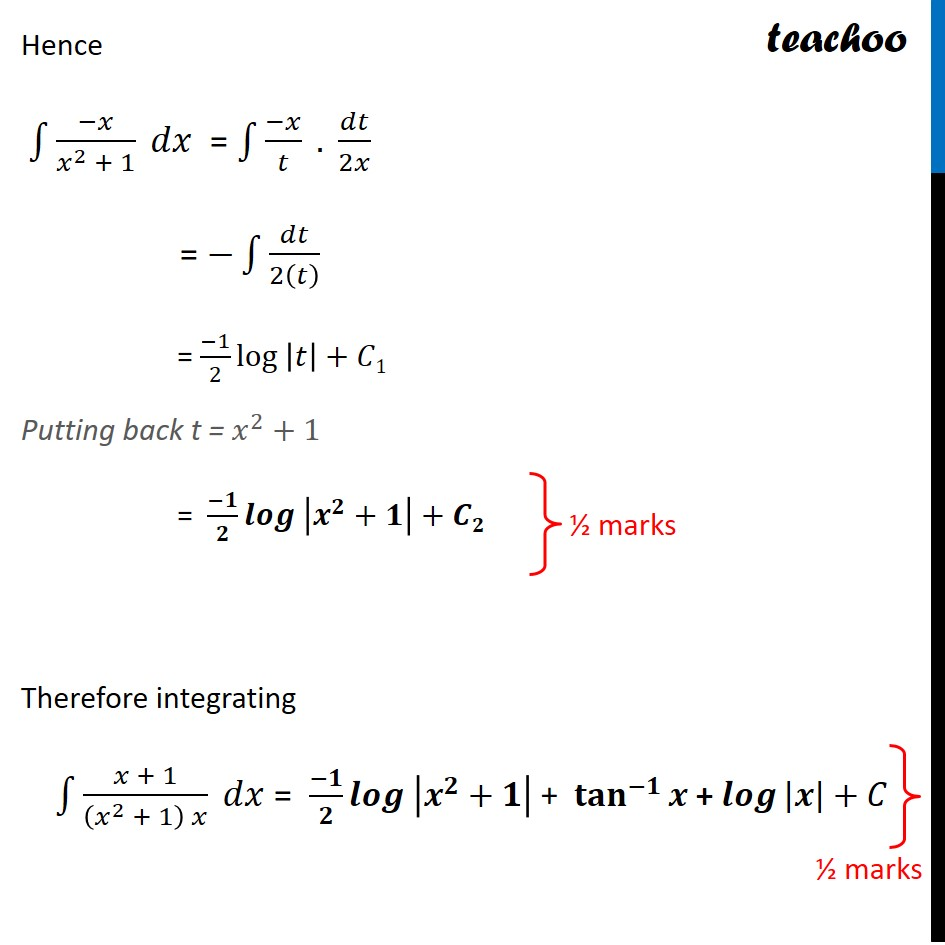Learn in your speed, with individual attention - Teachoo Maths 1-on-1 Class

### Transcript

Question 7 Find ∫1▒〖(𝑥 + 1)/((𝑥^2 + 1) 𝑥) 𝑑𝑥〗Let (𝑥 + 1)/((𝑥^2 + 1) 𝑥) = (𝑨𝒙 + 𝑩)/((𝒙^𝟐 + 𝟏) ) + 𝑪/𝒙 (𝑥 + 1)/((𝑥^2 + 1) 𝑥) = ((𝐴𝑥 + 𝐵)𝑥 + 𝐶(1 + 𝑥^2 ))/((𝑥^2 + 1) 𝑥) By cancelling denominator 𝒙 + 𝟏 = (𝑨𝒙 + 𝑩)𝒙 + 𝑪(𝟏 + 𝒙^𝟐 ) Putting 𝒙=𝟎 0 + 1 = (𝐴(0) + 𝐵) × 0 + 𝐶(1 +0^2 ) 1 = 𝐶 𝑪 = 𝟏 Putting 𝒙=𝟏 1 + 1 = (𝐴(1) + 𝐵) × 1 + 𝐶(1 +1^2 ) 2 = (𝐴 + 𝐵) +2𝐶 Putting 𝐶 = 1 2 = (𝐴 + 𝐵) +2 × 1 2 = (𝐴 + 𝐵) +2 2−2 = (𝐴 + 𝐵) 0 = 𝐴 + 𝐵 𝑨=− 𝑩 Putting 𝒙=−𝟏 −1 + 1 = (𝐴(−1) + 𝐵) × −1 + 𝐶(1 +〖(−1)〗^2 ) 0 = −(−𝐴 + 𝐵) +𝐶 × (1+1) 0 = −(−𝐴 + 𝐵) +2𝐶 Putting 𝐴=− 𝐵 0 = −(𝐵 + 𝐵) +2𝐶 0 = −2B +2𝐶 2B =2𝐶 B =𝐶 Putting 𝐶 = 1 𝑩 = 𝟏 And, 𝐴=−𝐵 ∴ 𝑨=−𝟏 Thus, 𝐴=−1, 𝐵=1, 𝐶 = 1 So, we can write (𝒙 + 𝟏)/((𝒙^𝟐 + 𝟏) 𝒙) = (𝐴𝑥 + 𝐵)/((𝑥^2 + 1) ) + 𝐶/𝑥 = ((−1)𝑥 +1)/((𝑥^2 + 1) ) + 1/𝑥 = (−𝒙 + 𝟏)/((𝒙^𝟐 + 𝟏) ) + 𝟏/𝒙 Therefore integrating ∫1▒(𝑥 + 1)/((𝑥^2 + 1) 𝑥) 𝑑𝑥 = ∫1▒(−𝒙 + 𝟏)/((𝒙^𝟐 + 𝟏) ) 𝑑𝑥 + ∫1▒1/(𝑥 ) 𝑑𝑥 = ∫1▒(−𝑥 + 1)/((𝑥^2 + 1) ) 𝑑𝑥 + ∫1▒1/(𝑥 ) 𝑑𝑥 = ∫1▒(−𝑥)/((𝑥^2 + 1) ) 𝑑𝑥 + ∫1▒1/(𝑥^2 + 1) 𝑑𝑥 + ∫1▒1/(𝑥 ) 𝑑𝑥 = ∫1▒(−𝑥)/((𝑥^2 + 1) ) 𝑑𝑥 + 〖𝐭𝐚𝐧〗^(−𝟏)⁡𝒙 + 𝒍𝒐𝒈⁡〖|𝒙|〗+𝐶 = ∫1▒(−𝑥)/((𝑥^2 + 1) ) 𝑑𝑥 + 〖𝐭𝐚𝐧〗^(−𝟏)⁡𝒙 + 𝒍𝒐𝒈⁡〖|𝒙|〗+𝐶 Solving 𝐈1 I1 = ∫1▒(−𝑥)/(𝑥^2 + 1) 𝑑𝑥 Let 𝒕 = 𝒙^𝟐+𝟏 𝑑𝑡/𝑑𝑥 = 2𝑥 𝑑𝑡/2𝑥 = 𝑑𝑥 Hence ∫1▒(−𝑥)/(𝑥^2 + 1) 𝑑𝑥 = ∫1▒〖(−𝑥)/𝑡 . 𝑑𝑡/2𝑥〗 = −∫1▒𝑑𝑡/2(𝑡) = (−1)/2 〖log 〗⁡|𝑡|+𝐶1 Putting back t = 𝑥^2+1 = (−𝟏)/𝟐 〖𝒍𝒐𝒈 〗⁡|𝒙^𝟐+𝟏|+𝑪𝟐 Therefore integrating ∫1▒(𝑥 + 1)/((𝑥^2 + 1) 𝑥) 𝑑𝑥 = (−𝟏)/𝟐 〖𝒍𝒐𝒈 〗⁡|𝒙^𝟐+𝟏| + 〖𝐭𝐚𝐧〗^(−𝟏)⁡𝒙 + 𝒍𝒐𝒈⁡〖|𝒙|〗+𝐶